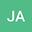loading page

A simple graph theoretic method provides accurate range area estimates
•• John Alroy
John Alroy
Macquarie University
Author Profile## Abstract

Calculating spatial ranges of species and individuals is a crucial problem throughout ecology. However, sample size biases can be strong, and defining range boundaries can be difficult. These hurdles can be overcome by calculating areas without calculating boundaries. The first step is to algorithmically define a graph that connects the spatial points where observations have been made. The routine generates a small number of short edges that form a pattern resembling a mosaic. The edge lengths are summed, squared, divided by the edge count, and multiplied by a known constant to obtain a total area estimate for the shape. This non-parametric mosaic area method can work with irregular outlines and clumped point distributions. It is more accurate than convex hull, kernel density, and hypervolume estimation according to simulation analyses. Mosaic area calculations can be used in areas ranging all the way from conservation biology to morphometrics.